# Hyperbolic functions

## Introduction

(I've written this topic specifically for students taking MEI FP2.)

The hyperbolic functions are a group of functions similar to the trigonometric functions. They're not periodic but they have a strong relationship to the exponential function.

This is the hyperbolic sine function, pronounced 'shine'

$$\sinh x = \frac{e^x -e^{-x}}{2}$$

The hyperbolic cosine function, pronounced 'cosh'

$$\cosh x = \frac{e^x +e^{-x}}{2}$$

The hyperbolic tangent function, pronounced 'than' or sometimes 'tanch'

$$\tanh x = \frac{\sinh x}{\cosh x} = \frac{e^x -e^{-x}}{e^x +e^{-x}}$$

Just like the $\cosec$, $\sec$, and $\cot$ functions you learned in C3, there are some equivalent hyperbolic functions. This is the hyperbolic cosecant function, defined for $x \ne 0$. It is pronounced 'cosech'

$$\csch x = \frac{1}{\sinh x} = \frac{2}{e^x-e^{-x}}$$

The hyperbolic secant function, pronounced 'sech'

$$\sech x = \frac{1}{\cosh x} = \frac{2}{e^x + e^{-x}}$$

The hyperbolic cotangent function, pronounced 'coth'

$$\coth x = \frac{1}{\tanh x} = \frac{e^x +e^{-x}}{e^x -e^{-x}}$$

## Graphs of hyperbolic functions

You need to be familiar with the graphs of the hyperbolic functions, and be able to sketch them.

This is the graph of $\sinh x$. It is equal to half the difference between $e^x$ and $e^{-x}$, and is an odd function.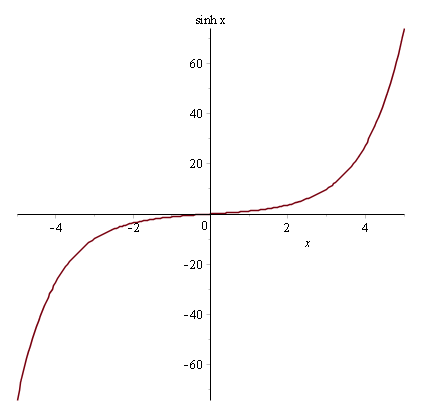This is the graph of $\cosh x$. It is equal to the arithmetic mean of $e^x$ and $e^{-x}$, and is an even function. The graph of $a\cosh(x/a)$ is called the catenary, the curve formed by a uniform string hanging freely between two fixed points under gravity.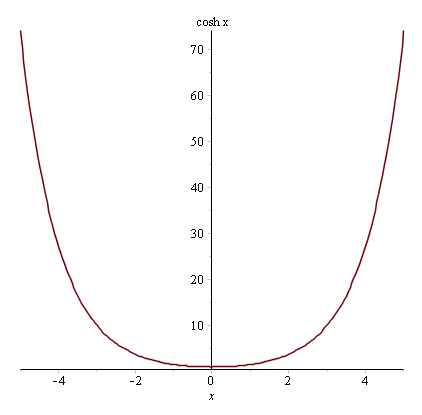This is the graph of $\tanh x$. It is an odd function.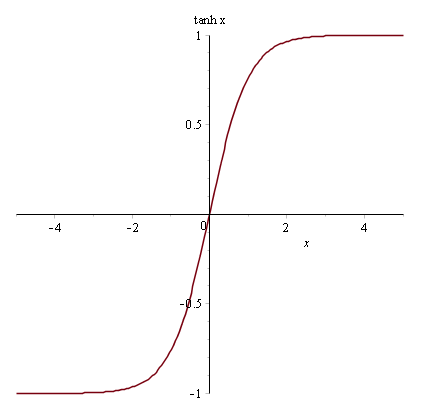This is the graph of $\csch x$. It is an odd function since $\sinh$ is also odd.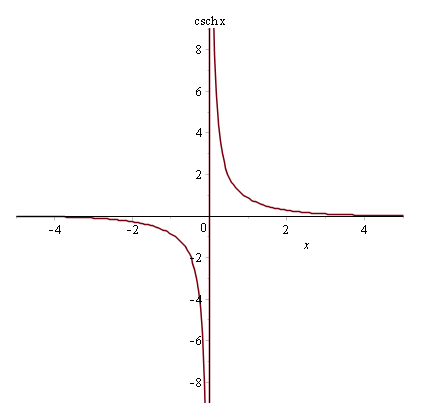This is the graph of $\sech x$. It is an even function since $\cosh$ is also even.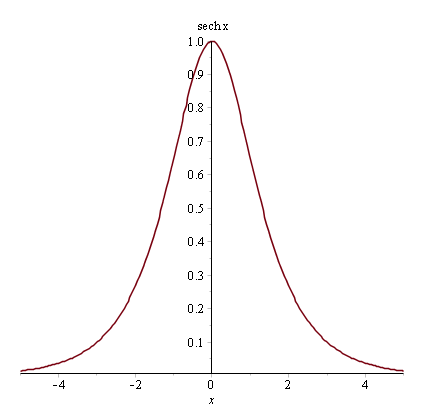And finally this is the graph of $\coth x$. It is an odd function since $\tanh$ is also odd.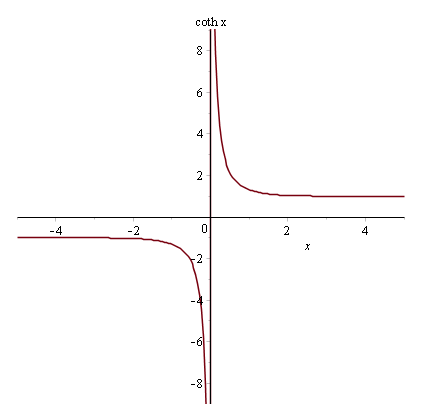## Solving equations with hyperbolic functions

In the exam you'll be asked to solve equations involving hyperbolic functions. Luckily most modern scientific calculators have a 'HYP' button that has hyperbolic functions preinstalled.

The hyperbolic functions are invertible, allowing you to solve equations like $\sinh x = 2$. The set of HYP functions on your calculator will include inverse hyperbolic functions so you could just find $x=\sinh^{-1} 2$. However you may be asked to find an exact solution by using their $e^x$ definitions.

### Example

Q) Find the exact solution of $\sinh x = 2$ using the $e^x$ definition of $\sinh$.

A) We have $$\sinh x = 2$$ Therefore $$\frac{e^x-e^{-x}}{2} = 2$$ Multiplying by $2e^x$ $$e^{2x}-1 = 4e^x \Rightarrow e^{2x}-4e^x-1=0$$ Solving for $e^x$ using the quadratic formula $$e^x = \frac{4\pm\sqrt{16+4}}{2} = 2\pm\sqrt{5}$$ But $e^x \gt 0$ so $$e^x = 2+\sqrt{5} \therefore x = \ln(2+\sqrt{5})$$

## Hyperbolic function identities

The hyperbolic functions have a series of curious identities that are very similar to the trig identities. In fact that's part of the reason why the hyperbolic functions are each named after a trig function.

$$\cosh^2 x - \sinh^2 x \equiv 1$$

\begin{align} \cosh^2 x - \sinh^2 x &= \left(\frac{e^x +e^{-x}}{2}\right)^2 - \left(\frac{e^x -e^{-x}}{2}\right)^2 \\ &= \frac{\left(e^x+e^{-x}\right)^2-\left(e^x-e^{-x}\right)^2}{4} \\ &= \frac{e^{2x}+2e^{x-x}+e^{-2x}-e^{2x}+2e^{x-x}-e^{-2x}}{4} \\ &= \frac{4}{4} = 1 \end{align}

Dividing through by $\cosh^2 x$ yields the next identity $$1-\tanh^2 x\equiv\sech^2 x$$

Instead dividing through by $\sinh^2 x$ yields the final identity $$\coth^2 x - 1\equiv\csch^2 x$$

## Osborn's rule

The similarities between the hyperbolic and trig functions are further highlighted by Osborn's rule which enables you to convert any trigonometric identity into a hyperbolic identity:

Replace sin with sinh and cos with cosh, but multiply any products of two sinh terms by -1

Indeed for the first identity I just showed you in the last section $$\cos^2 x + \sin^2 x \equiv 1 \to \cosh^2 x - \sinh^2 x \equiv 1$$

Since $\tanh = \sinh / \cosh$ it contains a $\sinh$ term $$1+\tan^2 x \equiv \sec^2 x \to 1-\tanh^2 x\equiv\sech^2 x$$ $$\cot^2 x +1\equiv\cosec^2 x \to \coth^2 x - 1\equiv\csch^2 x$$

For the trig addition formulae you learned in C3 $$\sin(A\pm B) = \sin A \cos B \pm \cos A \sin B \to \sinh(A \pm B) = \sinh A \cosh B \pm \cosh A \sinh B$$ $$\cos(A\pm B) = \cos A \cos B \mp \sin A \sin B \to \cosh(A\pm B) = \cosh A \cosh B \pm \sinh A \sinh B$$ $$\tan(A\pm B) = \frac{\tan A \pm \tan B}{1 \mp \tan A \tan B} \to \tanh(A\pm B) = \frac{\tanh A \pm \tanh B}{1 \pm \tanh A \tanh B}$$

Using Osborn's rule you can convert any trig identitiy into a hyperbolic identity.

## Inverse hyperbolic functions

Since the hyperbolic functions are one-to-one, there are inverse functions with simple formulae.

The formula for the inverse hyperbolic sine function is $$\sinh^{-1} x = \arsinh x = \ln(x+\sqrt{x^2+1})$$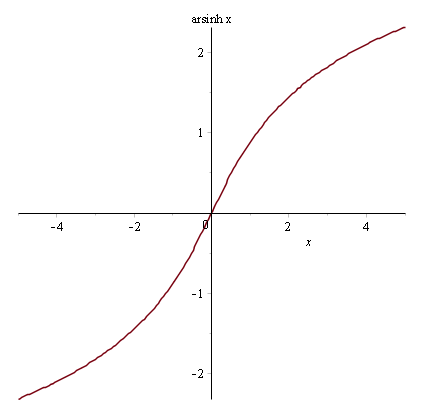The inverse hyperbolic cosine function, defined for $x \ge 1$ $$\cosh^{-1} x = \arcosh x = \ln(x+\sqrt{x^2-1})$$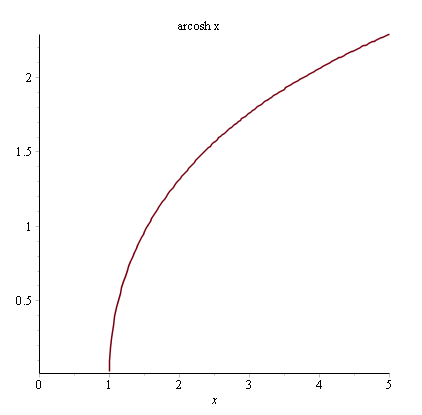The inverse hyperbolic tangent function, defined for $|x|\lt 1$ $$\tanh^{-1} x = \artanh x = \frac{1}{2}\ln\left(\frac{1+x}{1-x} \right)$$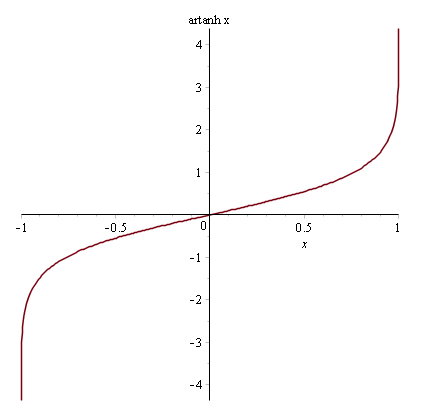The inverse hyperbolic cosecant function, defined for $x\ne 0$ $$\csch^{-1} x = \arcsch x = \ln\left(\frac{1}{x}+\frac{\sqrt{1+x^2}}{|x|} \right)$$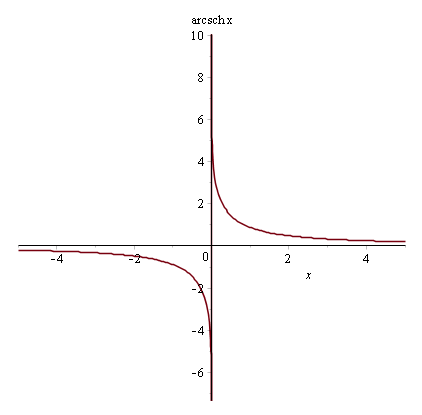The inverse hyperbolic secant function, defined for $x \in (0,1]$ $$\sech^{-1} x = \arsech x = \ln\left(\frac{1}{x}+\frac{\sqrt{1-x^2}}{|x|} \right)$$The inverse hyperbolic cotangent function, defined for $|x|\gt 1$ $$\coth^{-1} x = \arcoth x = \frac{1}{2}\ln\left(\frac{x+1}{x-1} \right)$$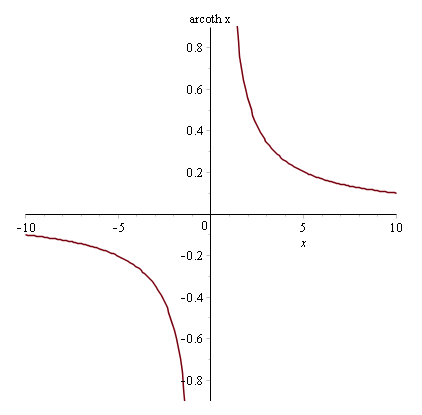### Example

Q) Solve $\sinh^2 x - \cosh x = 1$.

A) Using the identity $\cosh^2 x - \sinh^2 x \equiv 1$ and rearranging $$\left(\cosh^2 x - 1\right)-\cosh x - 1 = 0$$ $$\Rightarrow \cosh^2 x - \cosh x - 2 = 0$$ Then factorising $$\left(\cosh x+1\right)\left(\cosh x-2\right) = 0$$

So $\cosh x = -1,2$. However, $\cosh x \gt 0$ so the only working solution is $\cosh x = 2$. Therefore $$x = \arcosh 2 = \ln(2+\sqrt{2^2-1}) = \ln(2+\sqrt{3})$$

### Example

Q) Solve $\coth^2 x + 5\csch x + 5 = 0$.

A) Using the identity $\coth^2 x - 1 \equiv \csch^2 x$ $$\left(\csch^2 x + 1\right)+5\csch x + 5 = 0$$ $$\Rightarrow \csch^2 x +5\csch x + 6 = 0$$ Then factorising $$\left(\csch x+3\right)\left(\csch x+2\right)$$

So $\csch x = -3,-2$. Therefore $$x = \arcsch (-3) = \ln\left(\frac{1}{-3}+\frac{\sqrt{1+\left(-3\right)^2}}{|-3|}\right) = \ln\left(\frac{-1+\sqrt{10}}{3}\right)$$ Also $$x = \arcsch (-2) = \ln\left(\frac{1}{-2}+\frac{\sqrt{1+\left(-2\right)^2}}{|-2|}\right) = \ln\left(\frac{-1+\sqrt{5}}{2}\right)$$

### Example

Q) (Hard) Show that $\sech(2x) = \left(\frac{\tanh^2 x -1}{\tanh^2 x +1}\right)^2$.

A) First off we have the identity $1-\tanh^2 x\equiv\sech^2 x$ so $$\sech^2 (2x) = 1-\tanh^2 (2x)$$ By Osborn's rule we can find the identity $$\tanh(A+ B) = \frac{\tanh A + \tanh B}{1 + \tanh A \tanh B}$$ Therefore $$\tanh(2x) = \tanh(x+x) = \frac{\tanh x + \tanh x}{1 + \tanh x \tanh x} = \frac{2\tanh x}{1+\tanh^2 x}$$ Going back to the $\sech$ identity $$\sech^2 (2x) = 1-\tanh^2 (2x) = 1-\left(\frac{2\tanh x}{1+\tanh^2 x}\right)^2$$ Making a common denominator $$1 - \frac{4\tanh^2 x}{\left(1+\tanh^2 x\right)^2} = \frac{\left(1+\tanh^2 x\right)^2 - 4\tanh^2 x}{\left(1+\tanh^2 x\right)^2}$$ Expanding the square term in the numerator $$\frac{\left(1+\tanh^2 x\right)^2 - 4\tanh^2 x}{\left(1+\tanh^2 x\right)^2} = \frac{1+2\tanh^2 x + \tanh^4 x - 4\tanh^2 x}{\left(1+\tanh^2 x\right)^2}$$ Then finally simplifying the numerator and factorising the numerator $$\frac{\tanh^4 x - 2\tanh^2 x+1}{\left(1+\tanh^2 x\right)^2} = \frac{\left(\tanh^2 x -1\right)^2}{\left(\tanh^2 x +1\right)^2} = \left(\frac{\tanh^2 x -1}{\tanh^2 x +1}\right)^2$$

## Differentiating and integrating hyperbolic functions

The hyperbolic functions are fully differentiable, and you'll need to know how to differentiate and integrate various hyperbolic functions. In this section the striking similarities with the trig functions continue.

$$\frac{d}{dx}\sinh (ax) = a\cosh (ax)$$

$$\frac{d}{dx}\cosh (ax) = a\sinh (ax)$$

$$\frac{d}{dx}\tanh (ax) = a\sech^2 (ax)$$

$$\frac{d}{dx}\csch (ax) = -a\coth (ax)\csch (ax)$$

$$\frac{d}{dx}\sech (ax) = -a\tanh (ax)\sech (ax)$$

$$\frac{d}{dx}\coth (ax) = -a\csch^2 (ax)$$

### Example

Q) Differentiate $y=4\sinh^2 x$ with respect to $x$.

A) Let $y=u^2$ where $u = 2\sinh x$. Then using the chain rule $$\frac{dy}{dx}=\frac{dy}{du}\times\frac{du}{dx}=2u\left(2\cosh x\right) = 8\sinh x\cosh x$$

It's worth noting that by Osborn's rule this is equal to $4\sinh(2x)$.

### Example

Q) Differentiate $y=\frac{\tanh x}{x}$ with respect to $x$.

A) Let $u=\tanh x$ and $v=x$. Then by the quotient rule for differentiation $$\frac{dy}{dx}=\frac{vu'-uv'}{v^2} = \frac{x\sech^2 x-\tanh x}{x^2}$$

### Example

Q) Differentiate $y=\cosh(\sqrt{x})$ with respect to $x$.

A) Let $y=\cosh u$ where $u=\sqrt{x}$. Then using the chain rule $$\frac{dy}{dx}=\frac{dy}{du}\times\frac{du}{dx}=\sinh u \times \frac{1}{2}x^{-1/2} = \frac{\sinh(\sqrt{x})}{2\sqrt{x}}$$

These are the integrals for hyperbolic functions

$$\int \sinh (ax) \mathop{dx} = \frac{1}{a}\cosh(ax) +c$$

$$\int \cosh (ax) \mathop{dx} = \frac{1}{a}\sinh(ax) +c$$

$$\int \tanh (ax) \mathop{dx} = \frac{1}{a}\ln(\cosh(ax)) +c$$

$$\int \csch (ax) \mathop{dx} = \frac{1}{a}\ln\left|\cosh(ax)-\coth(ax)\right| +c$$

$$\int \sech (ax) \mathop{dx} = \frac{1}{a}\arctan(\sinh(ax)) +c$$

$$\int \coth (ax) \mathop{dx} = \frac{1}{a}\ln(\sinh(ax)) +c$$

Where $c$ is a constant of integration.

### Example

Q) Evaluate $\int_{0}^{3} \sinh(x/2) \mathop{dx}$ to one decimal place.

A) $$\int_{0}^{3} \sinh(x/2) \mathop{dx} = \left[2\cosh(x/2)\right]_{0}^{3} = 2\cosh(3/2)-2\cosh(0) = 2.7 \textrm{ units}^2 \textrm{ (1.d.p.)}$$

### Example

Q) Evaluate $\int_{0}^{1} x\cosh(x) \mathop{dx}$ to three significant figures.

A) We'll use integration by parts. Let $u=\sinh x$ and $v=x$ so that $u'=\cosh x$ and $v'=1$. \begin{align} \int u'v &= uv - \int uv' \\ \int_{0}^{1} x\cosh(x) \mathop{dx} &= \left[x\sinh x - \int\sinh x \mathop{dx} \right]_{0}^{1} \\ &= \left[x\sinh x - \cosh x\right]_{0}^{1} \\ &= \sinh (1) - \cosh (1) - 0 + \cosh (0) \\ &= 0.632 \textrm{ units}^2 \textrm{ (3.s.f.)} \end{align}

## Differentiating and integrating inverse hyperbolic functions

You also need to know how to differentiate three inverse hyperbolic functions.

$$\frac{d}{dx}\arsinh \left(\frac{x}{a}\right) = \frac{1}{\sqrt{x^2+a^2}}$$

$$\frac{d}{dx}\arcosh \left(\frac{x}{a}\right) = \frac{1}{\sqrt{x^2-a^2}}$$

$$\frac{d}{dx}\artanh \left(\frac{x}{a}\right) = \frac{a}{\sqrt{a^2-x^2}}$$

By knowing these derivatives of inverse hyperbolic functions you will be able to integrate certain functions. In the exam you might be asked to evaluate integrals of the form $$\frac{1}{\sqrt{x^2+a^2}} ~\textrm{ and }~ \frac{1}{\sqrt{x^2-a^2}}$$

Indeed $$\int \frac{1}{\sqrt{x^2+a^2}} \mathop{dx} = \arsinh \left(\frac{x}{a}\right) + c$$ $$\int \frac{1}{\sqrt{x^2-a^2}} \mathop{dx} = \arcosh \left(\frac{x}{a}\right) + c$$

where $c$ is a constant of integration.

### Example

Q) Evaluate $\int_{2}^{5} \frac{1}{\sqrt{4x^2+1}} \mathop{dx}$.

A) Factorise out 4 in the denominator $$\int_{2}^{5} \frac{1}{4x^2+1} \mathop{dx} = \int_{2}^{5} \frac{1}{\sqrt{4\left(x^2+\frac{1}{4}\right)}} \mathop{dx} = \frac{1}{2}\int_{2}^{5} \frac{1}{\sqrt{\left(x^2+\frac{1}{4}\right)}} \mathop{dx}$$ Now it's in standard form \begin{align} \frac{1}{2}\int_{2}^{5} \frac{1}{\sqrt{\left(x^2+\frac{1}{4}\right)}} \mathop{dx} = \frac{1}{2}\left[\arsinh (2x)\right]_{2}^{5} &= \frac{1}{2}\ln\left(10+\sqrt{10^2+1}\right)-\frac{1}{2}\ln\left(4+\sqrt{4^2+1}\right) \\ &= \frac{1}{2}\ln\left(\frac{10+\sqrt{101}}{4+\sqrt{17}}\right) \end{align}

### Example

Q) Evaluate $\int_{1}^{4}\frac{1}{\sqrt{x^2-4}} \mathop{dx}$.

A) $$\int_{1}^{4}\frac{1}{\sqrt{x^2-4}} \mathop{dx} = \left[\arcosh \left(\frac{x}{2}\right)\right]_{1}^{4} = \ln(2+\sqrt{2^2-1}) - 0 = \ln(2+\sqrt{3})$$